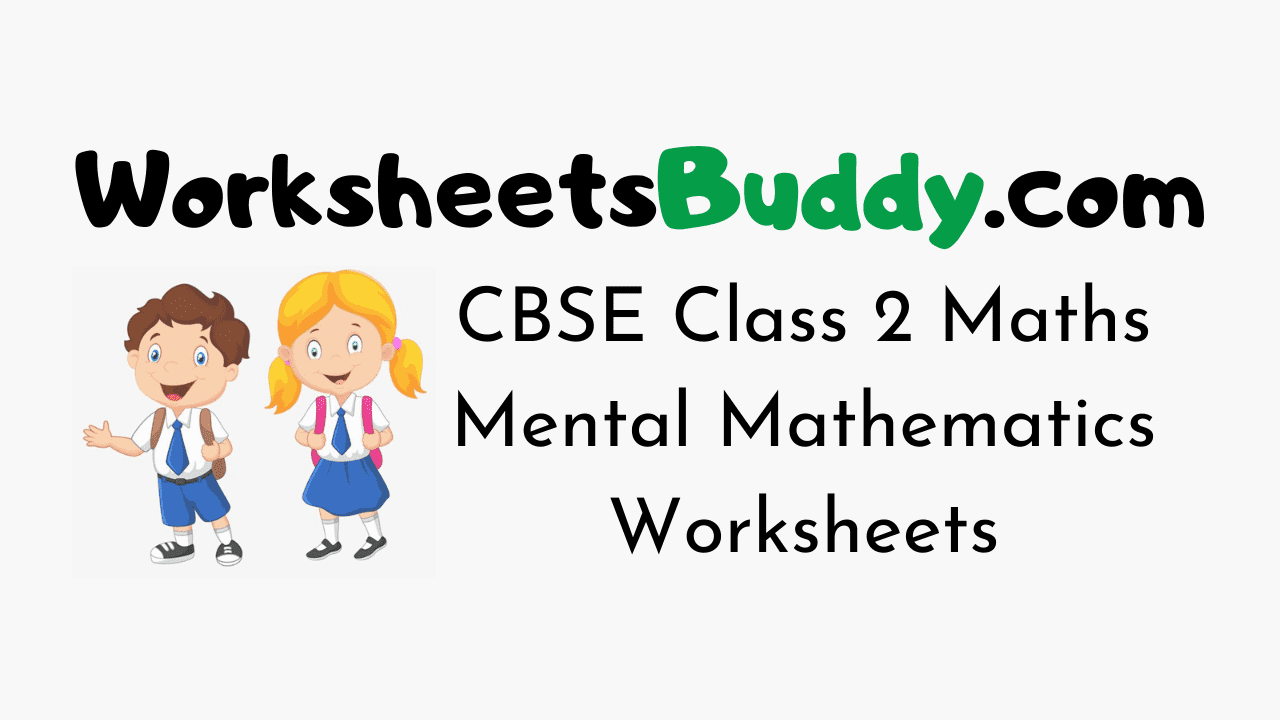# CBSE Class 2 Maths Mental Mathematics Worksheets

CBSE Class 2 Maths Mental Mathematics Worksheets – Worksheets have become an integral part and are the most engaging study materials for kids these days. The Standard 2 Maths Mental Mathematics Worksheets will help your younger one to practice what they have learned in class. Mental Mathematics Worksheets for Grade 2 will develop a curiosity in your kid to learn.

Maths Worksheets for Class 2 are a perfect combination of fun and learning. Find the most interesting and relevant puzzle activities for your kids here. In the Mathematics Grade 2 Mental Mathematics Worksheets Puzzles will be in the form of crosswords, true or false, pictures, mazes, fill-ups, MCQs, objectives, questions and answers, and so on.

Mathematics Worksheets for Class 2 on Mental Mathematics will help your kid remember what he/ she learned in school for a long time. Your Grade 2 kid will love to solve these engaging exercises and interesting collections of Puzzles.

### CBSE Class 2 Maths Mental Mathematics Worksheets

1. Complete the following: 15 subtract 4 is ______

2. Complete the following: 14 subtract 4 is ______

3. Complete the series: 0, 1, 2, 3, _______.

4. What is 10 more than 24?

5. What is 10 less than 16?

6. What is 10 less than 22?

7. Complete the series: 0, 25, 50, 75, ______.

8. What is 5 more than 10?

9. Write the numbers that comes next: 7, 14, 21, 28, _____.

10. Quickly add: 7 + 15

11. Complete the following: Double of 9 is ________

12. What is 5 more than 15?

13. Complete the following: 20 subtract 5 is ______

14. Quickly add: 6 + 4

15. Complete the following: 18 subtract 6 is ______

16. Is 24 x 9 = 218
A) True
B) False

17. Complete the following: 11 subtract 1 is ______

18. Quickly add: 2 + 15

19. Solve 58*52 = ?
A) 3016
B) 3220

20. Complete the following: 7 subtract 3 is ______

### CBSE Class 2 Maths Worksheet Answers

1. 11
2. 10
3. 4
4. 34
5. 6
6. 12
7. 100
8. 15
9. 35
10. 14
11. 18
12. 20
13. 15
14. 10
15. 12
16. Option B
17. 10
18. 17
19. Option A
20. 4### FAQs on Maths Mental Mathematics Class 2 Worksheets

1. How does a child learn Mathematics Mental Mathematics at Class 2 Level?

A child can learn Maths Mental Mathematics at Class 2 Level through the worksheets prevailing on our page.

2. What are the topics in Maths Class 2?

Mathematics Class 2 will have topics like Mental Mathematics etc.

3. Where can I get Mathematics Worksheets for Class 2 Mental Mathematics for free?

You can get Maths Mental Mathematics Worksheets Class 2 free of cost from our Worksheetsbuddy.com page. You can use them for your kid to get practice.

4. Are the CBSE Class 2 Mental Mathematics Worksheets over here printable?

Yes, the CBSE Class 2 Mental Mathematics Worksheets here are printable and you can download them easily.

Conclusion

We Hope the information prevailing on our page regarding CBSE Class 2 Mathematics Mental Mathematics Worksheets has been useful to you. For more info feel free to reach us and we will assist you at the easiest possible. Keep in touch with our site for more information on Class Wise Worksheets for various subjects.